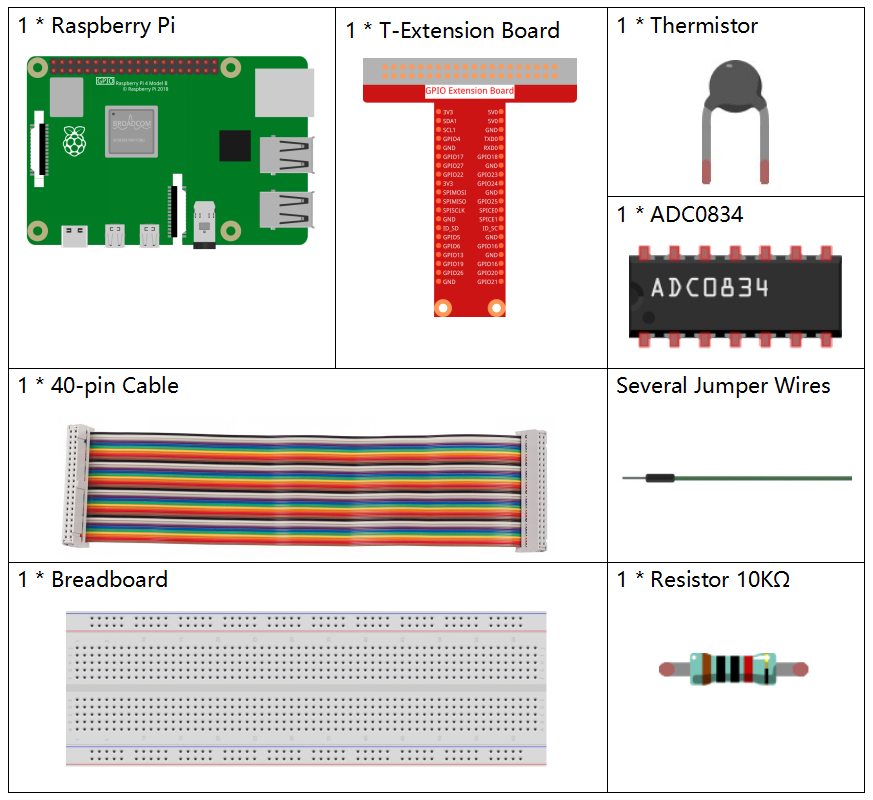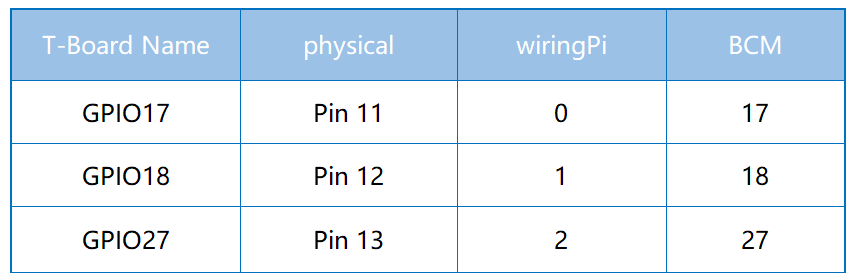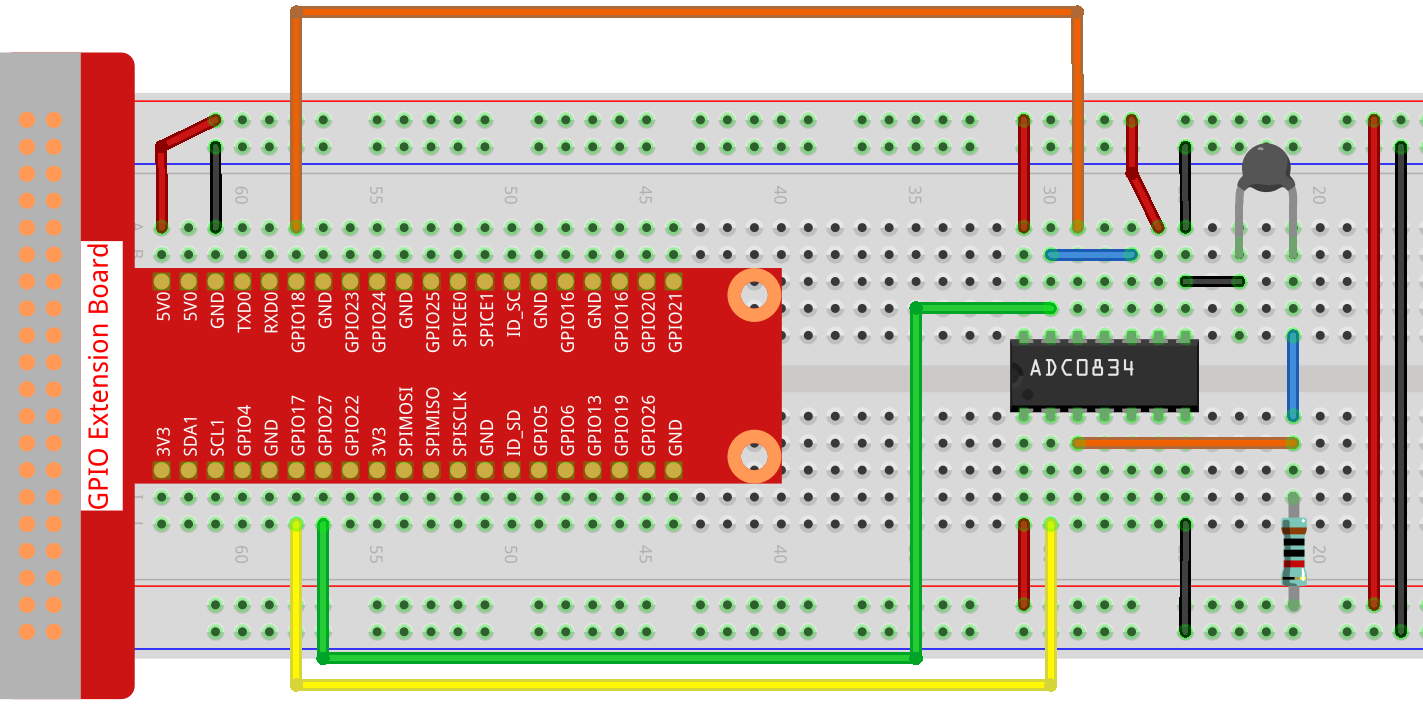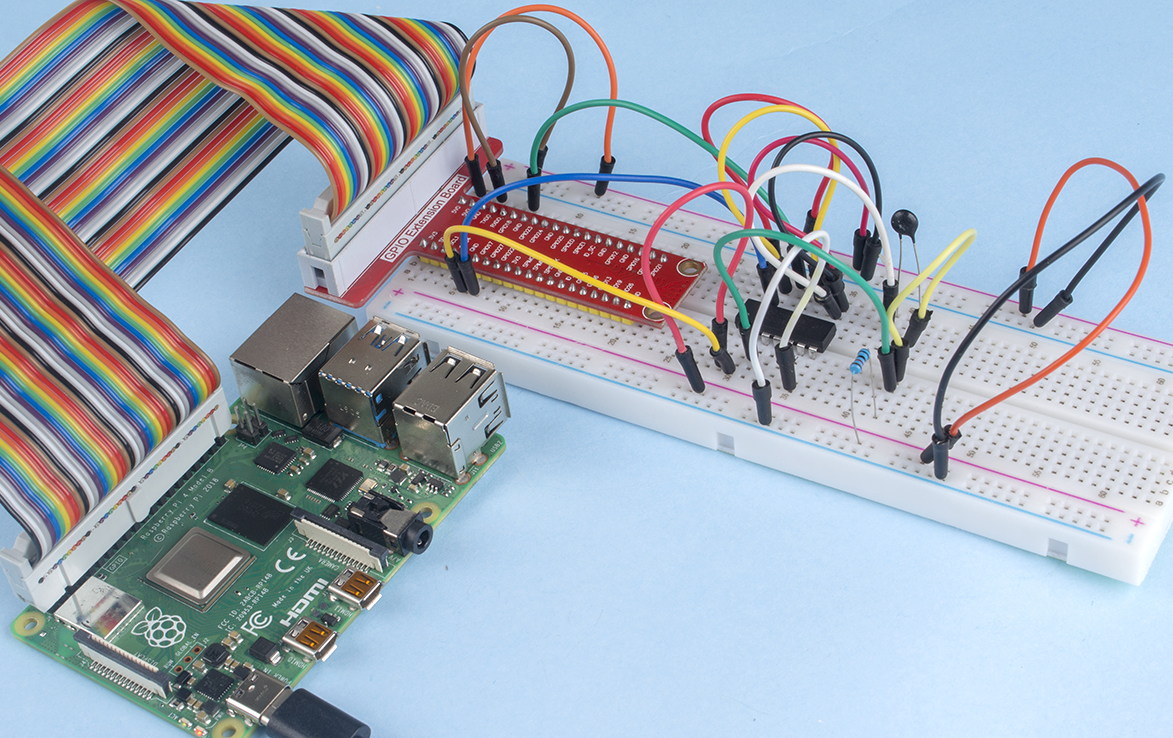# 2.2.2 Thermistor¶

## Introduction¶

Just like photoresistor can sense light, thermistor is a temperature sensitive electronic device that can be used for realizing functions of temperature control, such as making a heat alarm.

## Components¶## Schematic Diagram¶## Experimental Procedures¶

Step 1: Build the circuit.Step 2: Go to the folder of the code.

```cd /home/pi/raphael-kit/python/
```

Step 3: Run the executable file

```sudo python3 2.2.2_Thermistor.py
```

With the code run, the thermistor detects ambient temperature which will be printed on the screen once it finishes the program calculation.

Code

Note

You can Modify/Reset/Copy/Run/Stop the code below. But before that, you need to go to source code path like `raphael-kit/python`. After modifying the code, you can run it directly to see the effect.

```#!/usr/bin/env python3
# -*- coding: utf-8 -*-

import RPi.GPIO as GPIO
import time
import math

def init():

def loop():
while True:
Vr = 5 * float(analogVal) / 255
Rt = 10000 * Vr / (5 - Vr)
temp = 1/(((math.log(Rt / 10000)) / 3950) + (1 / (273.15+25)))
Cel = temp - 273.15
Fah = Cel * 1.8 + 32
print ('Celsius: %.2f °C  Fahrenheit: %.2f ℉' % (Cel, Fah))
time.sleep(0.2)

if __name__ == '__main__':
init()
try:
loop()
except KeyboardInterrupt:
```

Code Explanation

```import math
```

There is a numerics library which declares a set of functions to compute common mathematical operations and transformations.

```analogVal = ADC0834.getResult()
```

This function is used to read the value of the thermistor.

```Vr = 5 * float(analogVal) / 255
Rt = 10000 * Vr / (5 - Vr)
temp = 1/(((math.log(Rt / 10000)) / 3950) + (1 / (273.15+25)))
Cel = temp - 273.15
Fah = Cel * 1.8 + 32
print ('Celsius: %.2f °C  Fahrenheit: %.2f ℉' % (Cel, Fah))
```

These calculations convert the thermistor values into centigrade degree and Fahrenheit degree.

```Vr = 5 * float(analogVal) / 255
Rt = 10000 * Vr / (5 - Vr)
```

These two lines of codes are calculating the voltage distribution with the read value analog so as to get Rt (resistance of thermistor).

```temp = 1/(((math.log(Rt / 10000)) / 3950) + (1 / (273.15+25)))
```

This code refers to plugging Rt into the formula TK=1/(ln(RT/RN)/B+1/TN) to get Kelvin temperature.

```temp = temp - 273.15
```

Convert Kelvin temperature into centigrade degree.

```Fah = Cel * 1.8 + 32
```

Convert the centigrade degree into Fahrenheit degree.

```print ('Celsius: %.2f °C  Fahrenheit: %.2f ℉' % (Cel, Fah))
```

Print centigrade degree, Fahrenheit degree and their units on the display.

## Phenomenon Picture¶